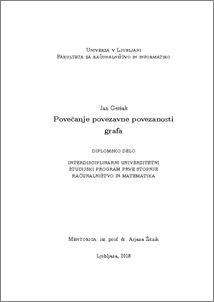# Edge-connectivity augmentation of a graph

Jan Geršak (2018) Edge-connectivity augmentation of a graph. EngD thesis.Preview
PDF

## Abstract

In this thesis we consider the edge-connectivity augmentation problem. In the first part of the thesis we present a cactus representation of a graph and describe its construction for which we present an algorithm. In the second part of the thesis we consider the relation between edge-connectivity of a graph and its cactus representation. Using this relation we give a lower bound for the least number of edges to be added to increase the edge-connectivity of a graph by one. We also prove that the lower bound is always achievable. Then we give an algorithm for edge-connectivity augmentation by one by applying properties of the cycle-type normal cactus representation. In the third part of the thesis we present general edge splitting method which is used in Frank's algorithm for solving edge-connectivity augmentation problem. We also prove Mader's theorem which is needed to prove finiteness of edge splitting in Frank's algorithm.

Item Type: Thesis (EngD thesis)
Keywords: graph, cactus representation, edge connectivity augmentation, edge splitting, Frank's algorithm, Mader's theorem.
Number of Pages: 53
Language of Content: Slovenian
Mentor / Comentors:
Name and SurnameIDFunction
izr. prof. dr. Arjana ŽitnikMentor